NEET  >  AIIMS Physics Mock Test - 6

# AIIMS Physics Mock Test - 6

Test Description

## 60 Questions MCQ Test AIIMS Mock Tests & Previous Year Papers | AIIMS Physics Mock Test - 6

AIIMS Physics Mock Test - 6 for NEET 2022 is part of AIIMS Mock Tests & Previous Year Papers preparation. The AIIMS Physics Mock Test - 6 questions and answers have been prepared according to the NEET exam syllabus.The AIIMS Physics Mock Test - 6 MCQs are made for NEET 2022 Exam. Find important definitions, questions, notes, meanings, examples, exercises, MCQs and online tests for AIIMS Physics Mock Test - 6 below.
Solutions of AIIMS Physics Mock Test - 6 questions in English are available as part of our AIIMS Mock Tests & Previous Year Papers for NEET & AIIMS Physics Mock Test - 6 solutions in Hindi for AIIMS Mock Tests & Previous Year Papers course. Download more important topics, notes, lectures and mock test series for NEET Exam by signing up for free. Attempt AIIMS Physics Mock Test - 6 | 60 questions in 60 minutes | Mock test for NEET preparation | Free important questions MCQ to study AIIMS Mock Tests & Previous Year Papers for NEET Exam | Download free PDF with solutions
 1 Crore+ students have signed up on EduRev. Have you?
AIIMS Physics Mock Test - 6 - Question 1

### A generator produces a voltage that is given by V=240 sin 120t where t is in sec. The frequency and r.m.s. voltage are

AIIMS Physics Mock Test - 6 - Question 2

### Suppose an electron is attracted towards the origin by a force k/r where 'k' is a constant and 'r' is the distance of the electron from the origin . By applying Bohr model to this system , the radius of the nth orbital of the electron is found to be 'r'n and the kinetic energy of the electron to be Tn . Then which of the following is true ?

AIIMS Physics Mock Test - 6 - Question 3

### A parallel plate capacitor is charged. If the plates are pulled apart

AIIMS Physics Mock Test - 6 - Question 4

A charged particle is released from rest in a region of steady and uniform electric and magnetic fields which are parallel to each other. The particle will move in a

AIIMS Physics Mock Test - 6 - Question 5

If momentum of a particle is doubled, then its de-Broglie's wavelength will

AIIMS Physics Mock Test - 6 - Question 6

A certain piece of silver of given mass is to be made like a wire. Which of the following combinations of length (L) and the area of cross-section (A) will lead to the smallest resistance

AIIMS Physics Mock Test - 6 - Question 7

A 2kg ball moving at 24 ms-1 undergoes inelastic head-on collision with a 4 kg ball moving in the opposite direction at 48 ms-1. If the coefficient of restitution is 2/3, their velocities in ms-1 after impact are

AIIMS Physics Mock Test - 6 - Question 8

Quantam nature of light is explained by which of the following phenomenon

AIIMS Physics Mock Test - 6 - Question 9

When yellow light is incident on a surface, no electrons are emitted while green light can emit. If red light is incident on the surface, then

AIIMS Physics Mock Test - 6 - Question 10

The coil of a dynamo is rotating in a magnetic field. The developed induced e.m.f. changes and the number of magnetic lines of force also changes. The phenomenon can be correctly described as

AIIMS Physics Mock Test - 6 - Question 11

Quantity that remains unchanged in a transformer is

AIIMS Physics Mock Test - 6 - Question 12

In an oscillating LC circuit, the maximum charge on the capacitor is Q. The charge on the capacitor, when the energy is stored equally between the electric and magnetic field is

AIIMS Physics Mock Test - 6 - Question 13

What is the value of inductance L for which the current is a maximum in series LCR circuit with C = 10 μF and ω = 1000s−1 ?

AIIMS Physics Mock Test - 6 - Question 14

The maximum distance upto which TV transmission from a TV tower of height h can be received is propotional to

AIIMS Physics Mock Test - 6 - Question 15

Which of the following represents the emf of a battery?

AIIMS Physics Mock Test - 6 - Question 16

A parallel plate capacitor is made by stacking n equally spaced plates connected alternatively. If capacitance between any two adjacent plates is C, then the resultant capacitance is

AIIMS Physics Mock Test - 6 - Question 17

A particle of mass m is moving in a horizontal circle of radius r under a centripetal force equal to - K/r2, where K is a constant. The total energy of the particle is

AIIMS Physics Mock Test - 6 - Question 18

If the unit of force and length be each increased by four times, then the unit of energy is increased by

AIIMS Physics Mock Test - 6 - Question 19

At temprature T, the poewr radiated by a body is Q watts. At the temprature 3T the power radiated by it will be

AIIMS Physics Mock Test - 6 - Question 20

A piece of fuse wire melts when a current of 15 ampere flows through it. With this current, if it dissipates 22.5 W, the resistance of fuse wire will be

AIIMS Physics Mock Test - 6 - Question 21

A bar magnet of pole strength 2 A-m is kept in a magnetic field of induction 4 x 10−5 wbm−2 such that the axis of the magnet makes an angle 30º with the direction of the field. The couple acting on the magnet is found to be 80 10−7N-m . Then the distance between the poles of the magnet is

AIIMS Physics Mock Test - 6 - Question 22

A bar magnet of magnetic moment M and length L is cut into two equal parts each of length L/2. The magnetic moment of each part will be

AIIMS Physics Mock Test - 6 - Question 23

How much force is required to produce an elongation of 0.2% in the length of a brass wire of diameter 0.6 mm? (Take Young's modulus for brass = 0.9x1011 N-m⁻2)

AIIMS Physics Mock Test - 6 - Question 24

A body is imparted motion rest to move in a straight line. If it is then obstructed by an opposite force, then

AIIMS Physics Mock Test - 6 - Question 25

Radioactive disintegration differs from a chemical change in being

AIIMS Physics Mock Test - 6 - Question 26

Two springs of constants k₁ and k₂, have equal highest velocities, when executing SHM. Then, the ratio of their amplitudes (given their masses are eqaul) will be

AIIMS Physics Mock Test - 6 - Question 27

In the following question, a Statement of Assertion (A) is given followed by a corresponding Reason (R) just below it. Read the Statements carefully and mark the correct answer-
Assertion(A): Electrical resistivity of typical insulator (≃ 1014Ω-m) is about 1022 times that of metal (≃ 10-8Ω-m) while in case of heat it is only about 103 (as KAg ≃ 400 W/mk while Kglass ≃ 1 W/mk)
Reason(R): In electrical conduction only free electrons take part while in thermal conduction both lattice and free electrons contribute.

AIIMS Physics Mock Test - 6 - Question 28

In the following question, a Statement of Assertion (A) is given followed by a corresponding Reason (R) just below it. Read the Statements carefully and mark the correct answer-
Assertion(A): When light goes from a medium of refraction index 1.5 to a medium of refraction index , 1.6, its speed decreases by 1.875 x 108 m/s.
Reason(R): Refraction index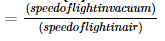Detailed Solution for AIIMS Physics Mock Test - 6 - Question 28

Speed of light in a medium of refractive index 1.5 is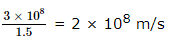Speed in a medium of refractive index 1.6 is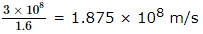∴ difference in speed = 2 x 108 - 1.875 x 108 = 1.25 x 107 m/s

AIIMS Physics Mock Test - 6 - Question 29

In the following question, a Statement of Assertion (A) is given followed by a corresponding Reason (R) just below it. Read the Statements carefully and mark the correct answer-
Assertion(A):A certain radioactive substance has a half life period of 30 days. Its disintegration is 0.0231 day.
Reason(R):Decay constant varies inversely as half life.

AIIMS Physics Mock Test - 6 - Question 30

In the following question, a Statement of Assertion (A) is given followed by a corresponding Reason (R) just below it. Read the Statements carefully and mark the correct answer-
Assertion(A): When net force is perpendicular to direction of velocity, it just changes its direction and not magnitude.
Reason (R): This results in uniform circular motion.

AIIMS Physics Mock Test - 6 - Question 31

In the following question, a Statement of Assertion (A) is given followed by a corresponding Reason (R) just below it. Read the Statements carefully and mark the correct answer-
Assertion(A): Orbiting satellite or body has K.E. of always less than that of Potential energy.
Reason(R): For any bound state, the magnitude of potential energy is always twice that of kinetic energy (K.E.)

AIIMS Physics Mock Test - 6 - Question 32

In the following question, a Statement of Assertion (A) is given followed by a corresponding Reason (R) just below it. Read the Statements carefully and mark the correct answer-
Assertion(A): In series LCR circuit, the resonance is equal and opposite to the capacitive reactance.
Reason (R): At resonance the inductive reactance is equal and opposite to the capacitive reactance.

AIIMS Physics Mock Test - 6 - Question 33

In the following question, a Statement of Assertion (A) is given followed by a corresponding Reason (R) just below it. Read the Statements carefully and mark the correct answer-
Assertion(A): Every oscillatory motion cannot be treated as simple harmonic, under small oscillation.
Reason(R): Even under larger oscillation restoring force should be proportional to displacement for being S.H.M.

AIIMS Physics Mock Test - 6 - Question 34

In the following question, a Statement of Assertion (A) is given followed by a corresponding Reason (R) just below it. Read the Statements carefully and mark the correct answer-
Assertion(A): When temperature of gas remains constant, speed of sound changes.
Reason(R) :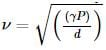( symbols have their usual meaning)

AIIMS Physics Mock Test - 6 - Question 35

In the following question, a Statement of Assertion (A) is given followed by a corresponding Reason (R) just below it. Read the Statements carefully and mark the correct answer-
Assertion(A): A current carrying wire produces electric field.
Reason(R): The current in a wire is due to the flow of free electrons in a definite direction.

AIIMS Physics Mock Test - 6 - Question 36

In the following question, a Statement of Assertion (A) is given followed by a corresponding Reason (R) just below it. Read the Statements carefully and mark the correct answer-
Assertion(A): An excess charge placed on an insulated conductor resides entirely on its outer surface.
Reason(R) : The excess charge placed inside a conductor will set up electrical fields insides the conductor which set up internal currents.

AIIMS Physics Mock Test - 6 - Question 37

In the following question, a Statement of Assertion (A) is given followed by a corresponding Reason (R) just below it. Read the Statements carefully and mark the correct answer-
Assertion(A): Everybody will have natural tendency to remain at rest.
Reason(R): Force always acts in the direction of motion.

AIIMS Physics Mock Test - 6 - Question 38

In the following question, a Statement of Assertion (A) is given followed by a corresponding Reason (R) just below it. Read the Statements carefully and mark the correct answer-
Assertion(A): Force between two parallel current carrying conductors is always of attraction.
Reason(R): Force per unit length =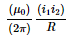(symbols have their usual meaning).

AIIMS Physics Mock Test - 6 - Question 39

In the following question, a Statement of Assertion (A) is given followed by a corresponding Reason (R) just below it. Read the Statements carefully and mark the correct answer-
Assertion(A): The direction of deflection of canal rays is the same as that of a stream of positively charged particles.
Reason(R): Canal rays cause fluoroscence when incident on certain materials.

Detailed Solution for AIIMS Physics Mock Test - 6 - Question 39

If the cathode of a discharge tube has holes in it, and pressure of the gas is around 1 mm of mercury, streams of faint luminous glow come out from each hole on the back side of the cathode. These are canal rays or positive rays. When molecules near the cathode are ionised the positive ions move slowly towards the cathode. The positive ions passing through the holes constitute the canal rays. Hence their direction of deflection is the same as that of a stream of positively changed particles

AIIMS Physics Mock Test - 6 - Question 40

In the following question, a Statement of Assertion (A) is given followed by a corresponding Reason (R) just below it. Read the Statements carefully and mark the correct answer-
Assertion(A):The path of γ-rays in Wilson's chamber are very thin continuous lines.
Reason(R):γ-rays produce very little ionisation.

AIIMS Physics Mock Test - 6 - Question 41

In the following question, a Statement of Assertion (A) is given followed by a corresponding Reason (R) just below it. Read the Statements carefully and mark the correct answer-
Assertion(A): Neutrons can be accelerated by cyclotron.
Reason(R): Neutrons are too heavy.

AIIMS Physics Mock Test - 6 - Question 42

In the following question, a Statement of Assertion (A) is given followed by a corresponding Reason (R) just below it. Read the Statements carefully and mark the correct answer-
Assertion(A): Out of galvanometer, ammeter and voltmeter resistance of ammeter is lowest and resistance of voltmeter is highest.
Reason(R): An ammeter is connected in series and a voltmeter is connected in parallel in a circuit.

AIIMS Physics Mock Test - 6 - Question 43

In the following question, a Statement of Assertion (A) is given followed by a corresponding Reason (R) just below it. Read the Statements carefully and mark the correct answer-
Assertion(A): The slope of work-time graph gives instantaneous power.
Reason(R): Instantaneous power is dot product of force and displacement.

AIIMS Physics Mock Test - 6 - Question 44

In the following question, a Statement of Assertion (A) is given followed by a corresponding Reason (R) just below it. Read the Statements carefully and mark the correct answer-
Assertion(A): A system of cylinder with non-accelerated motion of piston and larger temperature gradient is called a quasistatic process.
Reason(R) In quasi-static processes, the pressure and temperature of the surrounding and the cylinder are the same.

AIIMS Physics Mock Test - 6 - Question 45

In the following question, a Statement of Assertion (A) is given followed by a corresponding Reason (R) just below it. Read the Statements carefully and mark the correct answer-
Assertion(A): A charged particle undergoes non-rectilinear motion in a constant magnetic field. The only force acting on the particle is the magnetic force. Then kinetic energy of this particle remains constant but momentum of the particle changes.
Reason(R) : A force that always acts on the particle in direction perpendicular to its velocity does no work on the particle. But whenever a force acts on the particle, momentum of the particle changes.

AIIMS Physics Mock Test - 6 - Question 46

In the following question, a Statement of Assertion (A) is given followed by a corresponding Reason (R) just below it. Read the Statements carefully and mark the correct answer-
Assertion(A): There is a minimum wave-length below which no X-ray is emitted.
Reason(R): This wavelength depends on the material of the target.

AIIMS Physics Mock Test - 6 - Question 47

An electric motor exerts a force of 40 N on a cable and pulls it by a distance of 30 m in one minute. The power supplied by the motor is

AIIMS Physics Mock Test - 6 - Question 48

Two balls are projected making an angle of 30º and 45º respectively with the horizontal. If both have same velocity at the highest points of their paths, then the ratio of their velocities of projection is

Detailed Solution for AIIMS Physics Mock Test - 6 - Question 48

Given,
Angles of projection,
θ1 = 30º
θ2 = 45º
Suppose velocities of projection are u1 and u2
Now, at the highest point on the trajectory, the velocity of each ball is horizontal with components,
v1y = 0 = v2y
and v1x = u1 cos θ1
v2x = u2 cos θ2
According to question,
u1 cos θ1 = u2 cos θ2
u1 cos 30º = u2 cos 45º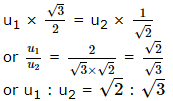AIIMS Physics Mock Test - 6 - Question 49

The colour of light wave that travels with maximum speed in glass is

AIIMS Physics Mock Test - 6 - Question 50

Band spectrum is formed due to

AIIMS Physics Mock Test - 6 - Question 51

Red light is used as danger signal because it is

AIIMS Physics Mock Test - 6 - Question 52

The typical ionisation energy of a donor in silicon is

AIIMS Physics Mock Test - 6 - Question 53

The dominant contribution to current comes from holes in case of

AIIMS Physics Mock Test - 6 - Question 54

Three equal resistors connected in series across a source of e.m.f. together dissipate 10 watt. If the same resistors are connected in parallel across the same e.m.f., then the power dissipated will be

AIIMS Physics Mock Test - 6 - Question 55

The unit of gravitational constant is

AIIMS Physics Mock Test - 6 - Question 56

'Parsec' is the unit of

AIIMS Physics Mock Test - 6 - Question 57

In a biprism expt., by using light of wavelength 5000 Å, 5 mm wide fringes are obtained on a screen 1.0 m away from coherent sources. The separation between two coherent sources is

AIIMS Physics Mock Test - 6 - Question 58

In a Young's experiment, two coherent sources are placed 0.90 mm apart and the fringes are observed one metre away. If it produces the second dark fringe at a distance of 1 mm from the central fringe, the wavelength of monochromatic light used would be

AIIMS Physics Mock Test - 6 - Question 59

In Young's double slit interference experiment the wavelength of light used is 6000 Å . If the path difference between waves reaching a point P on the screen is 1.5 microns, then at that point P

AIIMS Physics Mock Test - 6 - Question 60

A cord is used to lower vertically a block of mass M by a distance d with constant downward acceleration g/4. Work done by the cord on the block is

## AIIMS Mock Tests & Previous Year Papers

3 videos|41 docs|66 tests
 Use Code STAYHOME200 and get INR 200 additional OFF Use Coupon Code
Information about AIIMS Physics Mock Test - 6 Page
In this test you can find the Exam questions for AIIMS Physics Mock Test - 6 solved & explained in the simplest way possible. Besides giving Questions and answers for AIIMS Physics Mock Test - 6, EduRev gives you an ample number of Online tests for practice

## AIIMS Mock Tests & Previous Year Papers

3 videos|41 docs|66 tests

### How to Prepare for NEET

Read our guide to prepare for NEET which is created by Toppers & the best Teachers